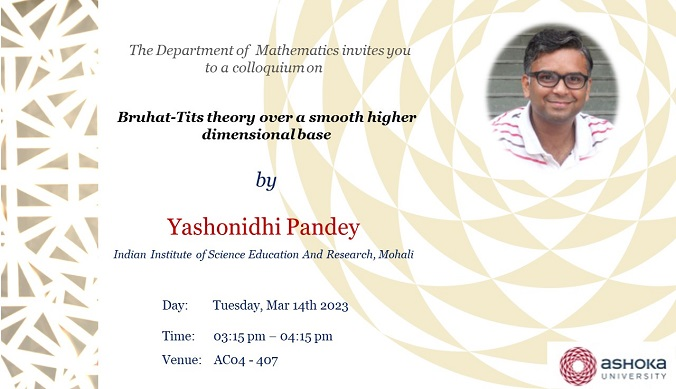# Event Calendar## Bruhat-Tits theory over a smooth higher dimensional base

Mathematics Colloquium | Yashonidhi Pandey | Mar 14th, 2023

• This event has passed.Abstract:

We report on a joint-work with Vikraman Balaji. It addresses the following question:

Let $G$ be an almost simple, split, simply-connected Chevalley group scheme over $\\mathbb{Z}$.

Let $\\mathbb{A}^\\circ$ denote the complement of the "axes" in $\\mathbb{A}^n_{_k}$.

Let $U$ be the union of $\\mathbb{A}^\\circ$ with $Spec$ of DVRs which are the local rings at the generic points of the axes in $\\mathbb{A}^n_{_k}$.

Given BT group schemes adapted to the axes divisors of $\\mathbb{A}^n_{_k}$,

we glue them with $G \\times \\mathbb{A}^\\circ$ to get a group scheme on $U$.

Does it extend to the whole space $\\mathbb{A}^n_{_k}$ as a flat group scheme?